# 【CV】第 3 章：使用 PyTorch 构建深度神经网络

🔎大家好，我是Sonhhxg_柒，希望你看完之后，能对你有所帮助，不足请指正！共同学习交流🔎

wcss

📝个人主页－Sonhhxg_柒的博客_CSDN博客 📃

QFusion

🎁欢迎各位→点赞👍 + 收藏⭐️ + 留言📝​

📣系列专栏 – 机器学习【ML】 自然语言处理【NLP】  深度学习【DL】LVGL

### 🖍foreword

✔说明⇢本人讲解主要包括Python、机器学习（ML）、深度学习（DL）、自然语言处理（NLP）等内容。

Date

pyecharts

slowfast

canvas

web网站

Ceph

rsync

Android图像识别

HashMap

uni.getLocation

L1 正则化

L2 正则化

• 表示图像
• 为什么要利用神经网络进行图像分析？
• 为图像分类准备数据
• 训练神经网络
• 缩放数据集以提高模型准确性
• 了解改变批量大小的影响
• 了解改变损失优化器的影响
• 了解改变学习率的影响
• 了解学习率热处理的影响
• 构建更深层次的神经网络
• 了解批量标准化的影响
• 过拟合的概念

# 表示图像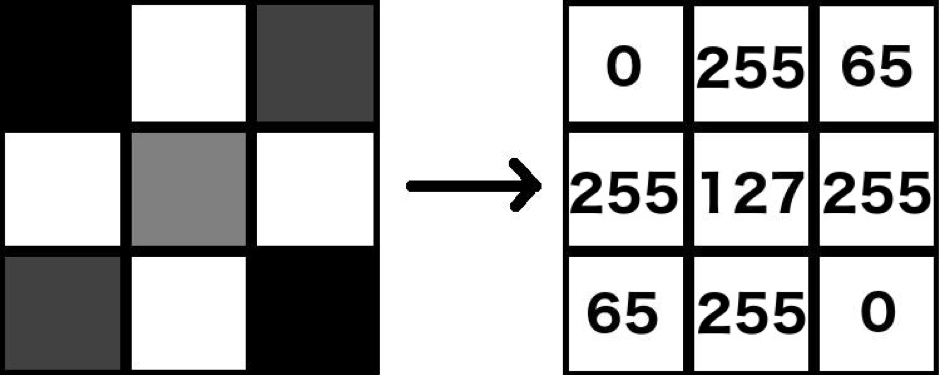## 将图像转换为结构化数组和标量

Python 可以将图像转换为结构化数组和标量，如下所示：

1.下载示例图像：

``！wget https://www.dropbox.com/s/l98leemr7r5stnm/Hemanvi.jpeg``

2.导入cv2（用于从磁盘读取图像）和matplotlib（用于绘制加载的图像）库并将下载的图像读入 Python 环境：

``````%matplotlib inline
import cv2, matplotlib.pyplot as plt

3.我们将在第 50-250 行以及第 40-240 列之间裁剪图像。最后，我们将使用以下代码将图像转换为灰度并绘制它：

``````# 裁剪图像
img = img[50:250,40:240]
# 将图像转换为灰度
img_gray = cv2.cvtColor(img, cv2.COLOR_BGR2GRAY)
# 显示图像
plt.imshow(img_gray, cmap='gray')``````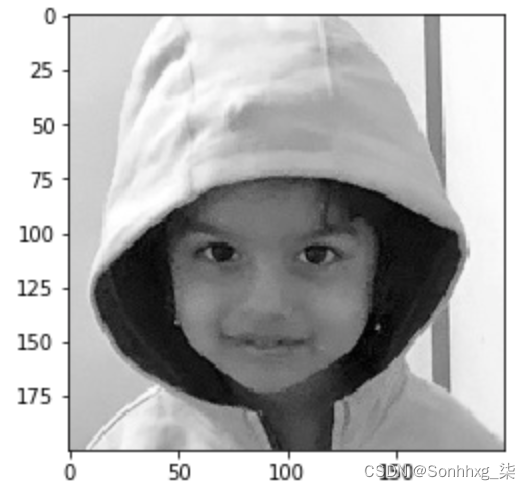您可能已经注意到前面的图像表示为 200 x 200 像素数组。现在，让我们减少用于表示图像的像素数量，以便我们可以在图像上叠加像素值（如果我们要在 200 x 200 数组上可视化像素值，这将更难做到） 25 x 25 阵列）。

4.将图像转换为 25 x 25 数组并绘制它：

``````img_gray_small = cv2.resize(img_gray,( 25 , 25 ))
plt.imshow(img_gray_small, cmap='gray')``````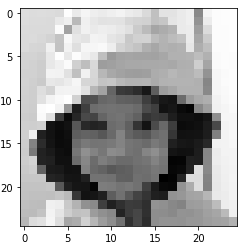5.让我们检查像素值。请注意，在以下输出中，由于空间限制，我们仅粘贴了前四行像素值：

``print(img_gray_small)``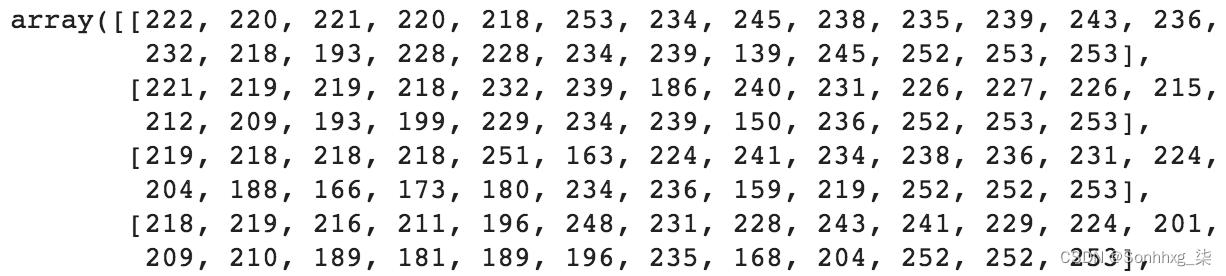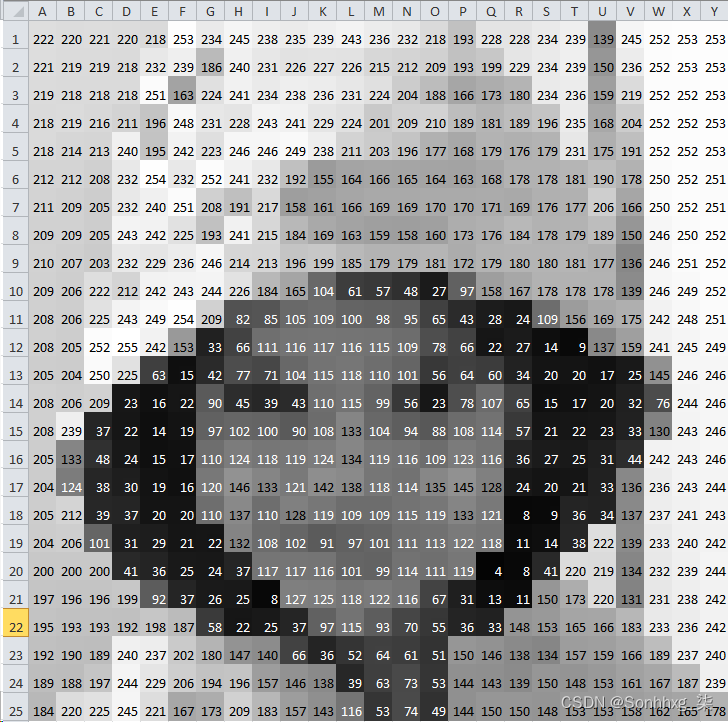1.下载彩色图像：

``！wget https://www.dropbox.com/s/l98leemr7r5stnm/Hemanvi.jpeg``

2.导入相关包并加载镜像：

``````import cv2, matplotlib.pyplot as plt
%matplotlib inline
img = cv2.imread( 'Hemanvi.jpeg' ) ``````

3.裁剪图像：

``````img = img[50:250,40:240,:]
img = cv2.cvtColor(img, cv2.COLOR_BGR2RGB) ``````

4.绘制获得的图像（请注意，如果您正在阅读印刷书籍并且尚未下载彩色图像包，它将以灰度显示）：

``````plt.imshow(img)
print(img.shape)
# (200,200,3)``````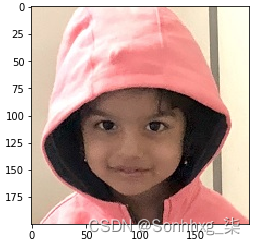5.右下角的 3 x 3 像素阵列可以通过如下方式获得：

``crop = img[-3:,-3:]``

6.打印并绘制像素值：

``````print(crop)
plt.imshow(crop)``````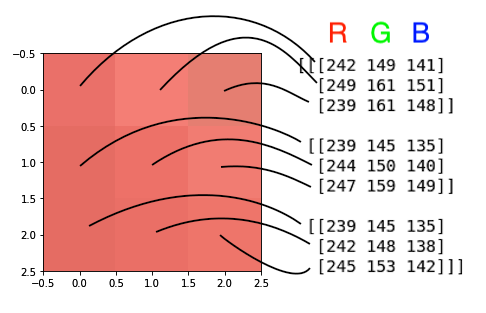# 为什么要利用神经网络进行图像分析？• Histogram feature（直方图特征）：对于一些任务，比如自动亮度或夜视，了解图片中的光照很重要；也就是说，亮或暗像素的比例。下图显示了示例图像的直方图。它描绘了图像被很好地照亮，因为在 255 处有一个尖峰：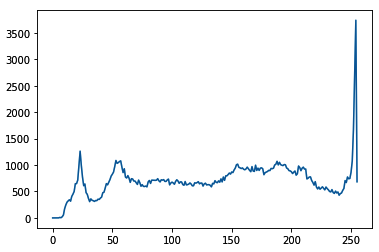• Edges and Corners feature（边缘和角落特征）：对于图像分割等任务，找到每个人对应的像素集很重要，首先提取边缘是有意义的，因为人的边界只是边缘的集合。在其他任务中，例如图像配准，检测关键地标至关重要。这些地标将是图像中所有角的子集。下图表示可以在我们的示例图像中找到的边缘和角落：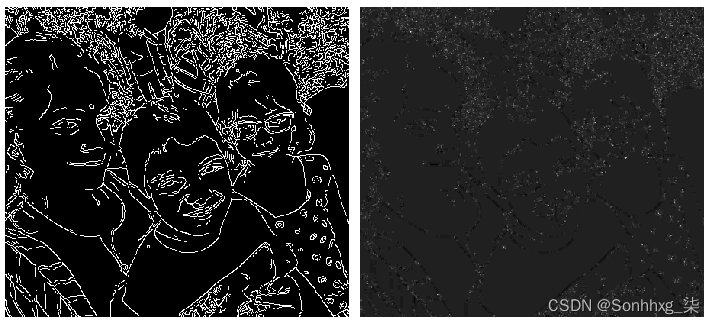• Color separation feature（颜色分离功能）：在自动驾驶汽车的交通信号灯检测等任务中，系统了解交通信号灯上显示的颜色非常重要。下图（最好以彩色方式查看）显示了示例图像的专用红色、绿色和蓝色像素：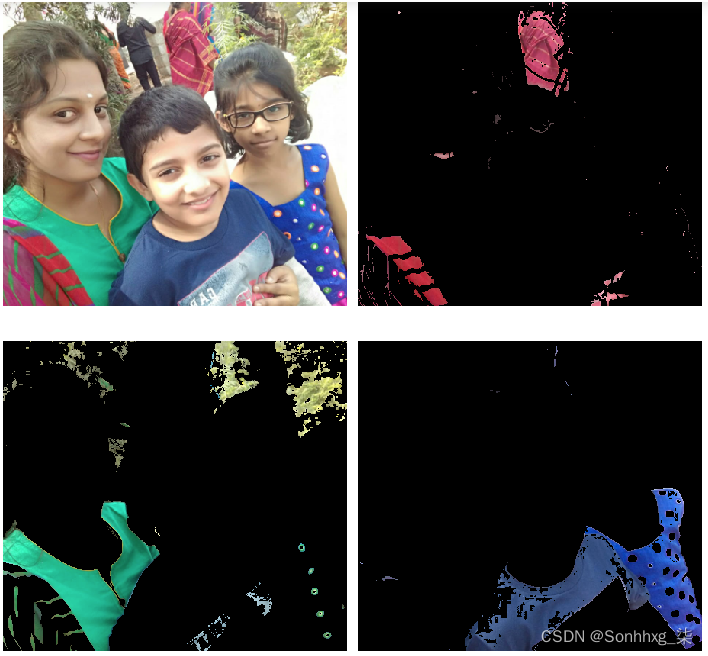• Image gradients feature（图像渐变 功能）：更进一步，了解颜色在像素级别如何变化可能很重要。不同的纹理可以给我们不同的梯度，这意味着它们可以用作纹理检测器。事实上，找到梯度是边缘检测的先决条件。下图显示了我们示例图像的一部分的整体梯度，以及梯度的 y 和 x 分量：这些只是其中的一小部分功能。还有很多，很难涵盖所有这些。创建这些特征的主要缺点是您需要成为图像和信号分析方面的专家，并且应该充分了解哪些特征最适合解决问题。即使满足这两个约束条件，也不能保证这样的专家能够找到正确的输入组合，即使找到了，也不能保证这样的组合在新的、看不见的场景中会起作用。

# 为图像分类准备我们的数据

1.首先下载数据集并导入相关包。该包包含各种数据集——其中一个是我们将在本章中处理的数据集： torchvision FashionMNIST

``````from torchvision import datasets
import torch
data_folder = '~/data/FMNIST' # 这可以是任何目录
# 你想下载 FMNIST 到
train=True)``````

• 接下来，我们必须存储 as 中可用的图像和fmnist.dataas中可用的tr_images标签（目标）：fmnist.targetstr_targets
``````tr_images = fmnist.data
tr_targets = fmnist.targets``````

2.检查我们正在处理的张量：

``````unique_values = tr_targets.unique()
print(f'tr_images & tr_targets:\n\tX -{tr_images.shape}\n\tY \
-{tr_targets.shape}\n\tY-Unique Values : {unique_values}')
print(f'UNIQUE CLASSES:\n\t{fmnist.classes}') ``````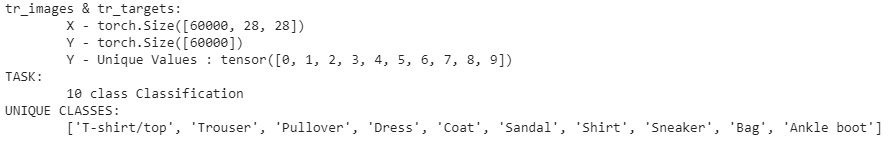3.为所有 10 个可能的类别绘制 10 个图像的随机样本：

• 导入相关包以绘制图像网格，以便您还可以处理 数组：
``````import matplotlib.pyplot as plt
%matplotlib inline
import numpy as np``````
• 创建一个图，我们可以在其中显示一个 10 x 10 网格，其中网格的每一行对应一个类，每一列呈现一个属于该行类的示例图像。循环遍历唯一的类号 ( ) 并获取对应于给定类号label_class的行索引 ( )：label_x_rows
``````R, C = len(tr_targets.unique()), 10
fig, ax = plt.subplots(R, C, figsize=(10,10))
for label_class, plot_row in enumerate(ax):
label_x_rows = np.where( tr_targets == label_class)``````

• 循环 10 次以填充给定行的列。此外，我们需要ix从与先前获得的给定类对应的索引中选择一个随机值 ( ) ( label_x_rows) 并绘制它们：
``````    for plot_cell in plot_row:
plot_cell.grid(False); plot_cell.axis('off')
ix = np.random.choice(label_x_rows)
x, y = tr_images[ix], tr_targets[ix]
plot_cell.imshow(x, cmap='gray')
plt.tight_layout()``````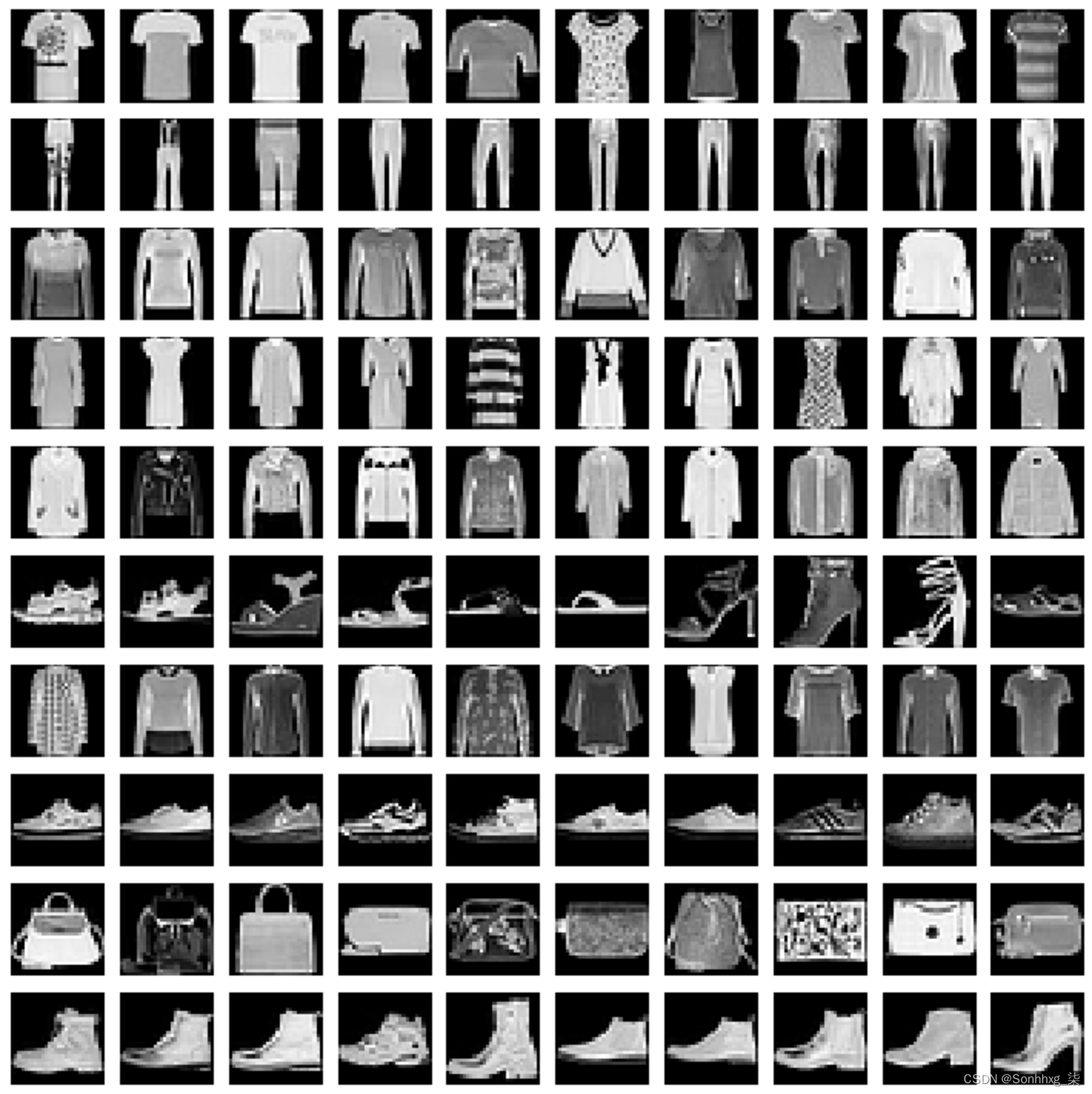# 训练神经网络

1. 导入相关包。
2. 构建一个可以一次获取一个数据点的数据的数据集。
4. 建立一个模型，然后定义损失函数和优化器。
5. 定义两个函数来分别训练和验证一批数据。
6. 定义一个函数来计算数据的准确性。
7. 在越来越多的时期根据每批数据执行权重更新。

1.导入相关包和 FMNIST 数据集：

``````from torch.utils.data import Dataset, DataLoader
import torch
import torch.nn as nn
import numpy as np
import matplotlib.pyplot as plt
%matplotlib inline
device = "cuda" if torch.cuda.is_available() else "cpu"
from torchvision import datasets
data_folder = '~/data/FMNIST' # This can be any directory you
train=True)
tr_images = fmnist.data
tr_targets = fmnist.targets``````

2.构建一个获取数据集的类。请记住，它是从一个类派生的，并且需要始终定义Dataset三个魔术函数—— __init__、__getitem__和—— ： __len__

``````class FMNISTDataset(Dataset):
def __init__(self, x, y):
x = x.float()
x = x.view(-1,28*28)
self.x, self.y = x, y
def __getitem__(self, ix):
x, y = self.x[ix], self.y[ix]
return x.to(device), y.to(device)
def __len__(self):
return len(self.x)``````

3.创建一个从数据集生成训练 DataLoader 的函数 trn_dl，称为FMNISTDataset. 这将为批量大小随机采样 32 个数据点：

``````def get_data():
train = FMNISTDataset(tr_images, tr_targets)
return trn_dl``````

4.定义一个模型，以及损失函数和优化器：

``````from torch.optim import SGD
def get_model():
model = nn.Sequential(
nn.Linear(28 * 28, 1000),
nn.ReLU(),
nn.Linear(1000, 10)
).to(device)
loss_fn = nn.CrossEntropyLoss()
optimizer = SGD(model.parameters(), lr=1e-2)
return model, loss_fn, optimizer``````

5.定义将在一批图像上训练数据集的函数：

``````def train_batch(x, y, model, opt, loss_fn):
model.train() # <- 让我们坚持下去，直到我们到达
# dropout 部分
# 像你批次上的任何 python 函数一样调用你的模型
# 输入
prediction = model(x)
# 计算损失
batch_loss = loss_fn(prediction, y)
# 基于 `model(x)` 中的前向传递计算所有
# 'model.parameters()' 的梯度
batch_loss.backward()
# 其中“f”是优化器
optimizer.step()
# 为下一批计算刷新梯度内存
return batch_loss.item()``````

6.构建一个计算给定 数据集准确性的函数：

``````# 因为不需要更新权重，
# 我们不妨不计算梯度。
# 在函数之上使用这个 '@' 装饰器
# 将禁用整个函数中的梯度计算
def accuracy(x, y, model):
model.eval() # <- 让我们等到dropout
# section
# 获取`x`图像张量的预测矩阵
prediction = model(x)
# 计算每行中最大值的位置是否
# coincides with ground truth
max_values, argmaxes = prediction.max(-1)
is_correct = argmaxes == y
return is_correct.cpu().numpy().tolist()``````

7.使用以下代码行训练神经网络：

• 初始化模型、损失、优化器和数据加载器：
``````trn_dl = get_data()
model, loss_fn, optimizer = get_model()``````
• 在每个 epoch 结束时调用包含准确率和损失值的列表：
``losses, accuracies = [], []``
• 定义时期数：
``````for epoch in range(5):
print(epoch)``````
• 调用将包含一个时期内每个批次对应的准确率和损失值的列表：
``    epoch_losses, epoch_accuracies = [], []``
``````    for ix, batch in enumerate(iter(trn_dl)):
x, y = batch``````
• 使用该函数训练批次，并将训练结束时的损失值存储在批次之上。此外，将不同批次的损失值存储在列表中： train_batch batch_lossepoch_losses
``````        batch_loss = train_batch(x, y, model, optimizer, \
loss_fn)
epoch_losses.append(batch_loss)``````
• 我们存储一个时期内所有批次的平均损失值：
``    epoch_loss = np.array(epoch_losses).mean()``
• 接下来，我们在所有批次的训练结束时计算预测的准确性：
``````    for ix, batch in enumerate(iter(trn_dl)):
x, y = batch
is_correct = accuracy(x, y, model)
epoch_accuracies.extend(is_correct)
epoch_accuracy = np.mean(epoch_accuracies)``````
• 将每个 epoch 结束时的损失和准确率值存储在列表中：
``````    losses.append(epoch_loss)
accuracies.append(epoch_accuracy)``````

``````epochs = np.arange(5)+1
plt.figure(figsize=(20,5))
plt.subplot(121)
plt.title('Loss value over increasing epochs')
plt.plot(epochs, losses, label='Training Loss')
plt.legend()
plt.subplot(122)
plt.title('Accuracy value over increasing epochs')
plt.plot(epochs, accuracies, label='Training Accuracy')
plt.gca().set_yticklabels(['{:.0f}%'.format(x*100) \
for x in plt.gca().get_yticks()])
plt.legend()``````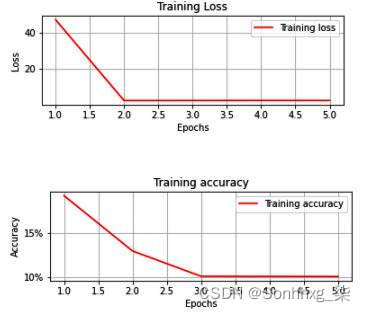# 缩放数据集以提高模型准确性

1.获取数据集，以及训练图像和目标，就像我们在上一节中所做的那样：

``````from torchvision import datasets
import torch
import torch.nn as nn
device = "cuda" if torch.cuda.is_available() else "cpu"
import numpy as np
data_folder = '~/data/FMNIST' # This can be any directory you
train=True)
tr_images = fmnist.data
tr_targets = fmnist.targets``````

2.修改，获取数据，使输入图像除以 255（像素的最大强度/值）： FMNISTDataset

``````class FMNISTDataset(Dataset):
def __init__(self, x, y):
x = x.float()/255
x = x.view(-1,28*28)
self.x, self.y = x, y
def __getitem__(self, ix):
x, y = self.x[ix], self.y[ix]
return x.to(device), y.to(device)
def __len__(self):
return len(self.x)``````

3.训练一个模型，就像我们在上一节的步骤 4567中所做的那样：

• 获取数据：
``````def get_data():
train = FMNISTDataset(tr_images, tr_targets)
return trn_dl``````
• 定义模型：
``````from torch.optim import SGD
def get_model():
model = nn.Sequential(
nn.Linear(28 * 28, 1000),
nn.ReLU(),
nn.Linear(1000, 10)
).to(device)
loss_fn = nn.CrossEntropyLoss()
optimizer = SGD(model.parameters(), lr=1e-2)
return model, loss_fn, optimizer``````
• 定义用于训练和验证一批数据的函数：
``````def train_batch(x, y, model, opt, loss_fn):
model.train()
# 调用你的模型，就像你的批处理上的任何 python 函数一样
# 输入
prediction = model(x)
# 计算损失
batch_loss = loss_fn(prediction, y)
# 基于 `model(x)` 中的前向传递计算所有
# 'model.parameters()' 的梯度
batch_loss.backward()
# apply new-weights = f(old-weights, old-weight-gradients)
#其中“f”是优化器
optimizer.step()
# 为下一批计算刷新内存
return batch_loss.item()
def accuracy(x, y, model):
model.eval ()
# 获取 `x` 图像张量的预测矩阵
prediction = model(x)
# 计算每行中最大值的位置是否
# 与地面实况一致
max_values, argmaxes = prediction.max(-1)
is_correct = argmaxes == y
return is_correct.cpu().numpy().tolist()``````
• 在越来越多的时期训练模型：
``````trn_dl = get_data()
model, loss_fn, optimizer = get_model()
losses, accuracies = [], []
for epoch in range(5):
print(epoch)
epoch_losses, epoch_accuracies = [], []
for ix, batch in enumerate(iter(trn_dl)):
x, y = batch
batch_loss = train_batch(x, y, model, optimizer,
loss_fn)
epoch_losses.append(batch_loss)
epoch_loss = np.array(epoch_losses).mean()
for ix, batch in enumerate(iter(trn_dl)):
x, y = batch
is_correct = accuracy(x, y, model)
epoch_accuracies.extend(is_correct)
epoch_accuracy = np.mean(epoch_accuracies)
losses.append(epoch_loss)
accuracies.append(epoch_accuracy)``````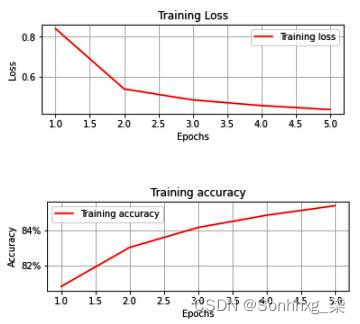在下表中，我们根据前面的公式计算了Sigmoid列：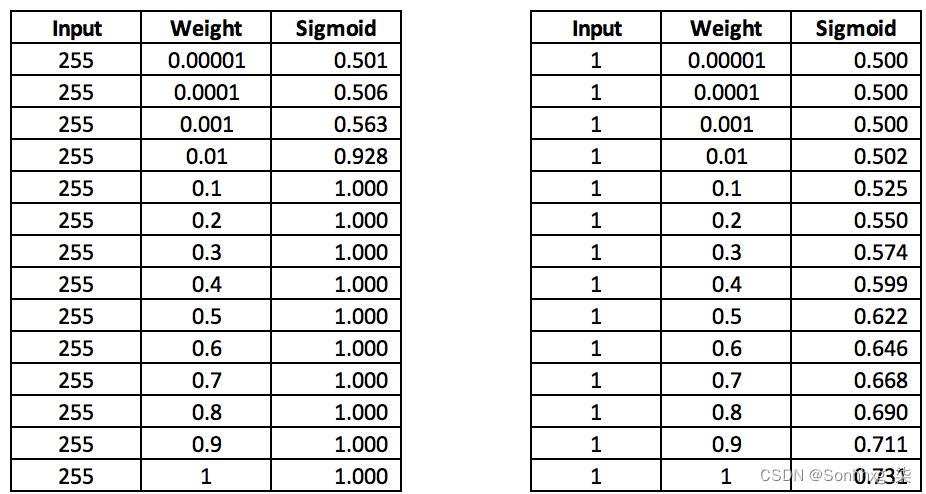# 了解改变批量大小的影响

• 当训练批量大小为 32 时，训练和验证数据的损失和准确度值。
• 当训练批量大小为 10,000 时，训练和验证数据的损失和准确度值。

## 批量大小 32

1.下载并导入训练图像和目标：

``````from torchvision import datasets
import torch
data_folder = '~/data/FMNIST' # This can be any directory you
train=True)
tr_images = fmnist.data
tr_targets = fmnist.targets``````

2.与训练图像类似，我们必须通过在数据集中调用方法train = False时指定来下载和导入验证数据集FashionMNIST：

``````val_fmnist =datasets.FashionMNIST(data_folder,download=True, \
train=False)
val_images = val_fmnist.data
val_targets = val_fmnist.targets``````

3.导入相关包并定义device：

``````import matplotlib.pyplot as plt
%matplotlib inline
import numpy as np
import torch
import torch.nn as nn
device = 'cuda' if torch.cuda.is_available() else 'cpu'``````

4.定义数据集类 ( FashionMNIST)、用于训练一批数据的函数 ( train_batch)、计算准确度 ( accuracy)，然后定义模型架构、损失函数和优化器 ( get_model)。请注意，获取数据的函数将是唯一与我们在前面部分中看到的函数有偏差的函数（因为我们现在正在处理训练和验证数据集），因此我们将在下一步中构建它：

``````class FMNISTDataset(Dataset):
def __init__(self, x, y):
x = x.float()/255
x = x.view(-1,28*28)
self.x, self.y = x, y
def __getitem__(self, ix):
x, y = self.x[ix], self.y[ix]
return x.to(device), y.to(device)
def __len__(self):
return len(self.x)

def get_model():
model = nn.Sequential(
nn.Linear(28 * 28, 1000),
nn.ReLU(),
nn.Linear(1000, 10)
).to(device)

loss_fn = nn.CrossEntropyLoss()
return model, loss_fn, optimizer

def train_batch(x, y, model, opt, loss_fn):
model.train()
prediction = model(x)
batch_loss = loss_fn(prediction, y)
batch_loss.backward()
optimizer.step()
return batch_loss.item()

def accuracy(x, y, model):
model.eval()
# this is the same as @torch.no_grad
# at the top of function, only difference
# being, grad is not computed in the with scope
prediction = model(x)
max_values, argmaxes = prediction.max(-1)
is_correct = argmaxes == y
return is_correct.cpu().numpy().tolist()``````

5.定义一个将获取数据的函数；也就是说，get_data. 此函数将返回批大小为 32 的训练数据和批大小为验证数据长度的验证数据集（我们不会使用验证数据来训练模型；我们只会使用它来了解模型的未见数据的准确性）：

``````def get_data():
train = FMNISTDataset(tr_images, tr_targets)
val = FMNISTDataset(val_images, val_targets)
shuffle=False)
return trn_dl, val_dl``````

6.定义一个计算验证数据损失的函数；也就是说，. 请注意，我们是单独计算的，因为在训练模型时会计算训练数据的损失： val_loss

``````@torch.no_grad()
def val_loss(x, y, model):
model.eval()
prediction = model(x)
val_loss = loss_fn(prediction, y)
return val_loss.item()``````

``````trn_dl, val_dl = get_data()
model, loss_fn, optimizer = get_model()``````

8.训练模型，如下：

• 初始化包含训练和验证准确性的列表，以及增加时期的损失值：
``````train_losses, train_accuracies = [], []
val_losses, val_accuracies = [], []``````
• 循环五个时期并初始化包含给定时期内各批训练数据的准确性和损失的列表：
``````for epoch in range(5):
print(epoch)
train_epoch_losses, train_epoch_accuracies = [], []``````
• 遍历一批训练数据并计算一个 epoch 内的准确率 ( train_epoch_accuracy) 和损失值 ( )：train_epoch_loss
``````    for ix, batch in enumerate(iter(trn_dl)):
x, y = batch
batch_loss = train_batch(x, y, model, optimizer, \
loss_fn)
train_epoch_losses.append(batch_loss)
train_epoch_loss = np.array(train_epoch_losses).mean()

for ix, batch in enumerate(iter(trn_dl)):
x, y = batch
is_correct = accuracy(x, y, model)
train_epoch_accuracies.extend(is_correct)
train_epoch_accuracy = np.mean(train_epoch_accuracies)``````
• 计算一批验证数据内的损失值和准确率（因为验证数据的批大小等于验证数据的长度）：
``````    for ix, batch in enumerate(iter(val_dl)):
x, y = batch
val_is_correct = accuracy(x, y, model)
validation_loss = val_loss(x, y, model)
val_epoch_accuracy = np.mean(val_is_correct)``````

• 最后，我们将训练和验证数据集的准确度和损失值附加到包含时代级别聚合验证和准确度值的列表中。我们这样做是为了在下一步中查看 epoch 级别的改进：
``````    train_losses.append(train_epoch_loss)
train_accuracies.append(train_epoch_accuracy)
val_losses.append(validation_loss)
val_accuracies.append(val_epoch_accuracy)``````

9.可视化训练和验证数据集中的准确性和损失值在增加的 时期内的改进：

``````epochs = np.arange(5)+1
import matplotlib.ticker as mtick
import matplotlib.pyplot as plt
import matplotlib.ticker as mticker
%matplotlib inline
plt.subplot(211)
plt.plot(epochs, train_losses, 'bo', label='Training loss')
plt.plot(epochs, val_losses, 'r', label='Validation loss')
plt.gca().xaxis.set_major_locator(mticker.MultipleLocator(1))
plt.title('Training and validation loss \
when batch size is 32')
plt.xlabel('Epochs')
plt.ylabel('Loss')
plt.legend()
plt.grid('off')
plt.show()
plt.subplot(212)
plt.plot(epochs, train_accuracies, 'bo', \
label='Training accuracy')
plt.plot(epochs, val_accuracies, 'r', \
label='Validation accuracy')
plt.gca().xaxis.set_major_locator(mticker.MultipleLocator(1))
plt.title('Training and validation accuracy \
when batch size is 32')
plt.xlabel('Epochs')
plt.ylabel('Accuracy')
plt.gca().set_yticklabels(['{:.0f}%'.format(x*100) \
for x in plt.gca().get_yticks()])
plt.legend()
plt.grid('off')
plt.show()``````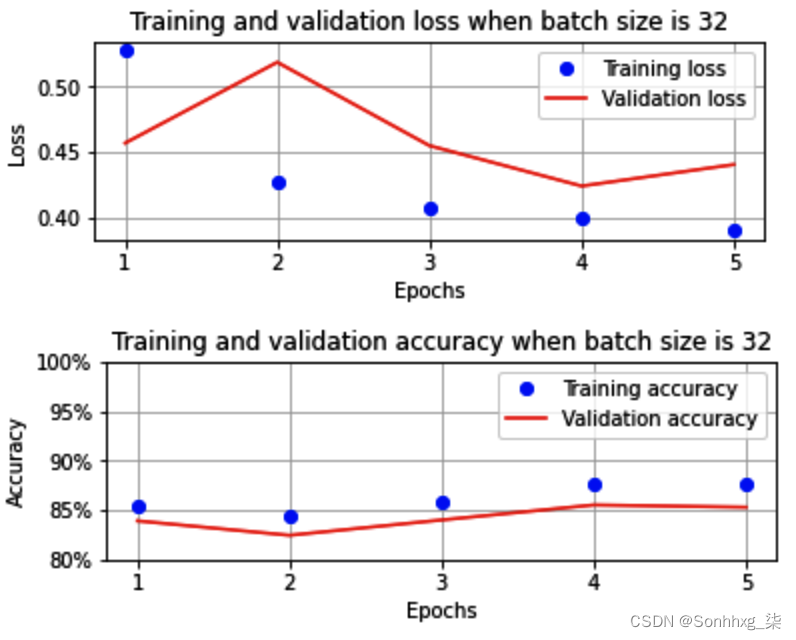如您所见，当批大小为 32 时，到五个 epoch 结束时，训练和验证的准确率约为 85%。接下来，我们batch_size 将在 get_data函数中训练 DataLoader 时改变参数batch_size ，看看它对最后准确率的影响五个时代。

## 批量大小为 10,000

``````def get_data():
train = FMNISTDataset(tr_images, tr_targets)
val = FMNISTDataset(val_images, val_targets)
shuffle=False)
return trn_dl, val_dl``````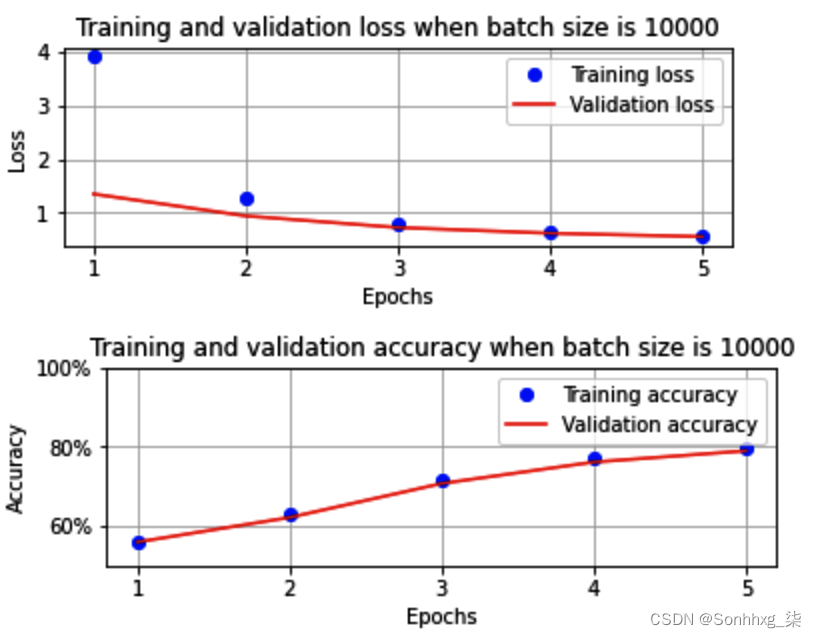# 了解改变损失优化器的影响

• 修改优化器，使其成为随机梯度下降SGD ) 优化器
• 在 DataLoader 中获取数据时恢复为 32 的批处理大小
• 将 epoch 数增加到 10（以便我们可以比较 SGD 和 Adam 在更长的 epoch 数上的性能）

1.修改优化器，以便在函数中使用 SGD 优化器，get_model 同时确保其他所有内容保持不变：

``````from torch.optim import SGD, Adam
def get_model():
model = nn.Sequential(
nn.Linear(28 * 28, 1000),
nn.ReLU(),
nn.Linear(1000, 10)
).to(device)

loss_fn = nn.CrossEntropyLoss()
optimizer = SGD(model.parameters(), lr=1e-2)
return model, loss_fn, optimizer``````

2.增加我们将用于训练模型的 epoch 数：

``````train_losses, train_accuracies = [], []
val_losses, val_accuracies = [], []
for epoch in range(10):
train_epoch_losses, train_epoch_accuracies = [], []
for ix, batch in enumerate(iter(trn_dl)):
x, y = batch
batch_loss = train_batch(x, y, model, optimizer, \
loss_fn)
train_epoch_losses.append(batch_loss)
train_epoch_loss = np.array(train_epoch_losses).mean()

for ix, batch in enumerate(iter(trn_dl)):
x, y = batch
is_correct = accuracy(x, y, model)
train_epoch_accuracies.extend(is_correct)
train_epoch_accuracy = np.mean(train_epoch_accuracies)

for ix, batch in enumerate(iter(val_dl)):
x, y = batch
val_is_correct = accuracy(x, y, model)
validation_loss = val_loss(x, y, model)
val_epoch_accuracy = np.mean(val_is_correct)

train_losses.append(train_epoch_loss)
train_accuracies.append(train_epoch_accuracy)
val_losses.append(validation_loss)
val_accuracies.append(val_epoch_accuracy)``````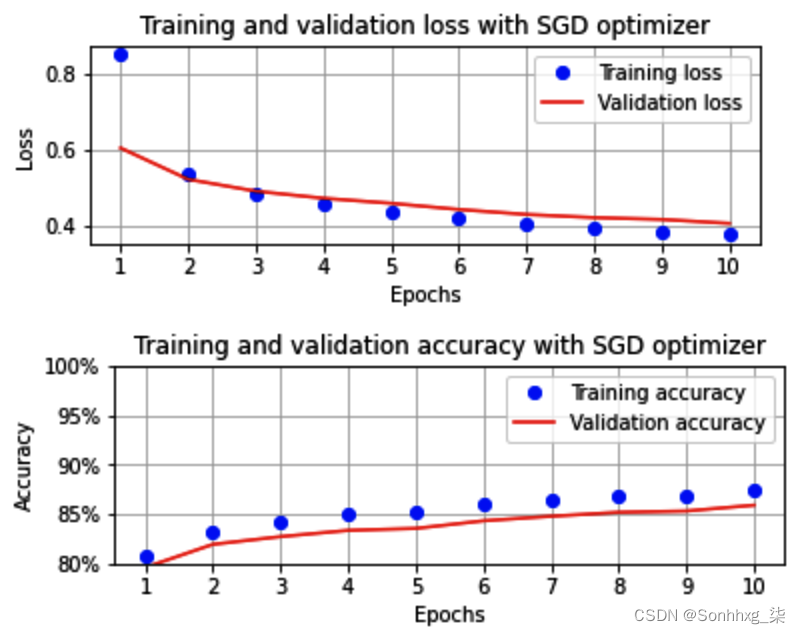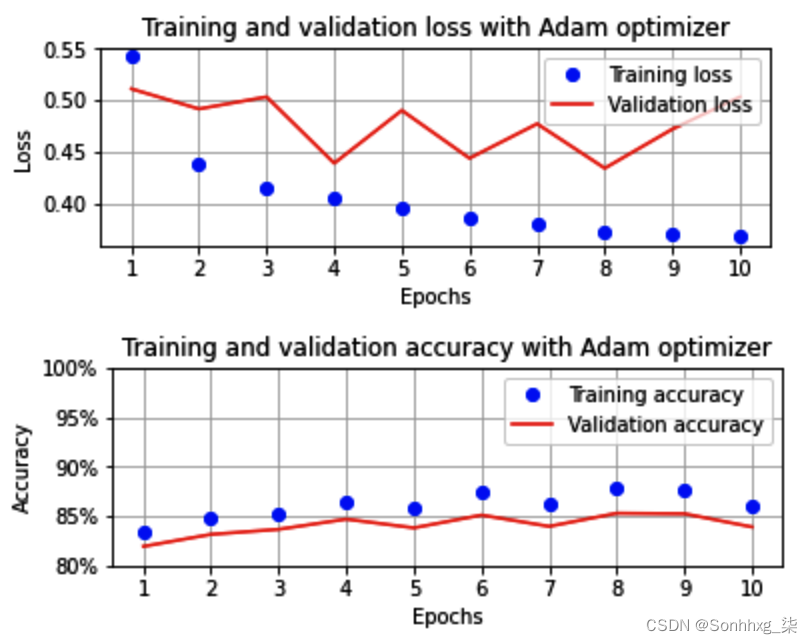# 了解改变学习率的影响

• 缩放数据集上的更高学习率 (0.1)
• 缩放数据集上的较低学习率 (0.00001)
• 在非缩放数据集上降低学习率 (0.001)
• 非缩放数据集上的更高学习率 (0.1)

## 学习率对缩放数据集的影响

• 高学习率
• 中等学习率
• 学习率低

### 高学习率

• 当我们使用 Adam 优化器时，我们需要执行的步骤将与Batch size of 32部分完全相同。
• 唯一的变化optimizer是我们定义get_model函数时的学习率。在这里，我们将学习率 (lr ) 更改为 0.1。

``````def get_model():
model = nn.Sequential(
nn.Linear(28 * 28, 1000),
nn.ReLU(),
nn.Linear(1000, 10)
).to(device)

loss_fn = nn.CrossEntropyLoss()
return model, loss_fn, optimizer``````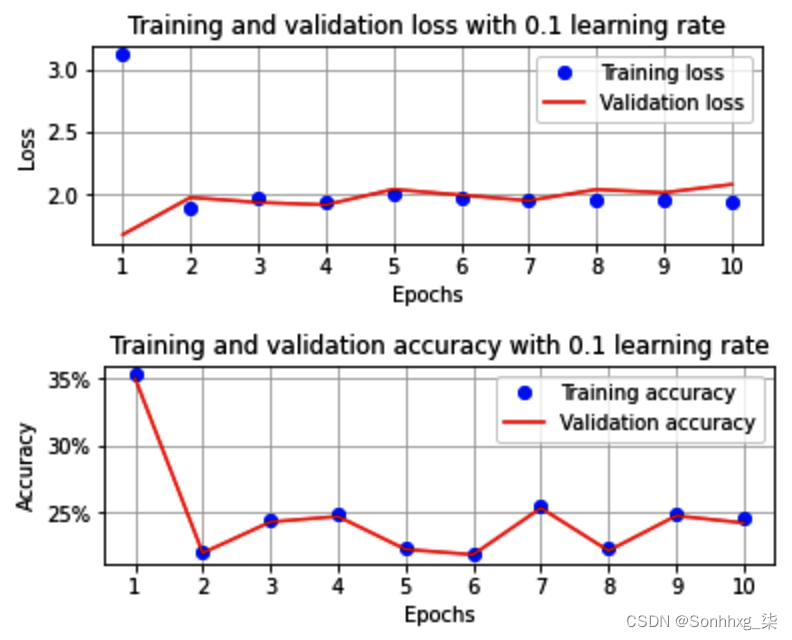### 中等学习率

``````def get_model():
model = nn.Sequential(
nn.Linear(28 * 28, 1000),
nn.ReLU(),
nn.Linear(1000, 10)
).to(device)

loss_fn = nn.CrossEntropyLoss()
return model, loss_fn, optimizer ``````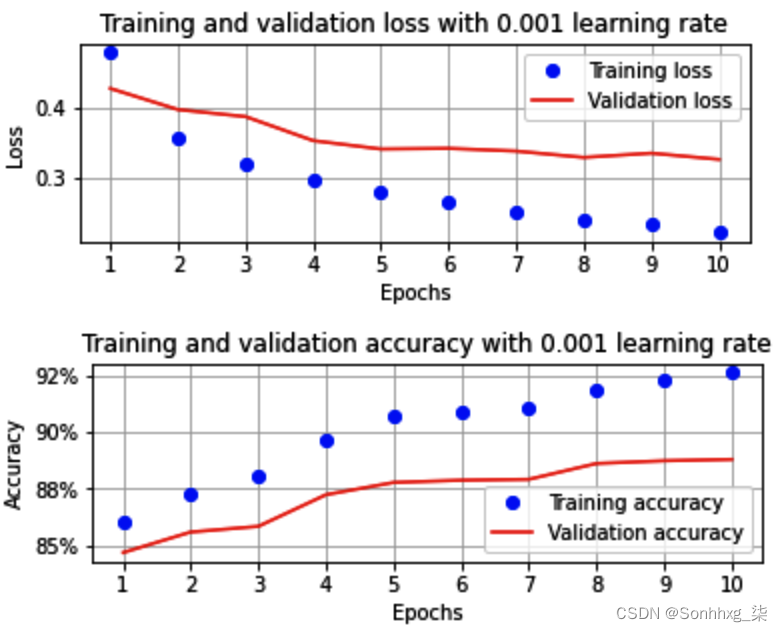### 学习率低

``````def get_model():
model = nn.Sequential(
nn.Linear(28 * 28, 1000),
nn.ReLU(),
nn.Linear(1000, 10)
).to(device)

loss_fn = nn.CrossEntropyLoss()
return model, loss_fn, optimizer ``````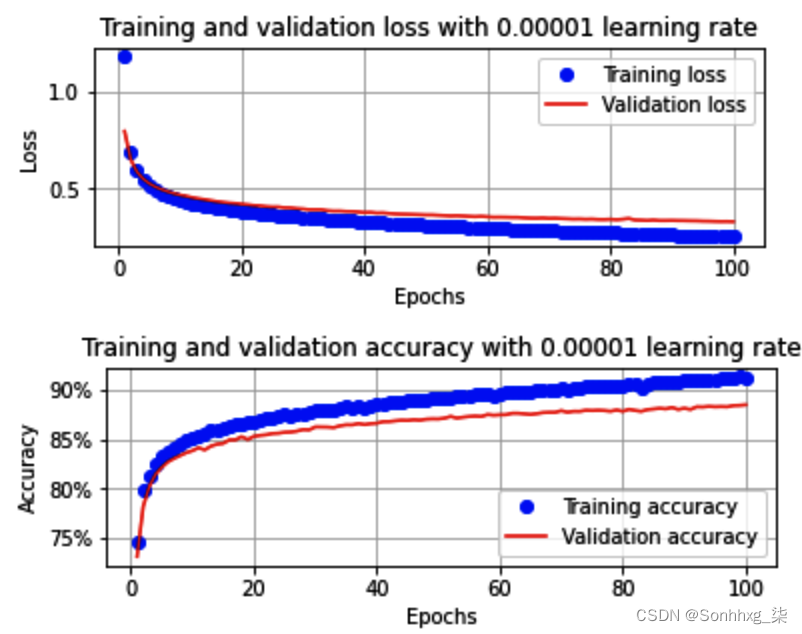### 不同学习率的跨层参数分布

• 连接输入层和隐藏层的层中的权重
• 隐藏层中的偏差
• 连接隐藏层和输出层的层中的权重
• 输出层的偏差

``````for ix, par in enumerate(model.parameters()):
if(ix==0):
plt.hist(par.cpu().detach().numpy().flatten())
plt.title('Distribution of weights conencting \
input to hidden layer')
plt.show()
elif(ix ==1):
plt.hist(par.cpu().detach().numpy().flatten())
plt.title('Distribution of biases of hidden layer')
plt.show()
elif(ix==2):
plt.hist(par.cpu().detach().numpy().flatten())
plt.title('Distribution of weights conencting \
hidden to output layer')
plt.show()
elif(ix ==3):
plt.hist(par.cpu().detach().numpy().flatten())
plt.title('Distribution of biases of output layer')
plt.show()``````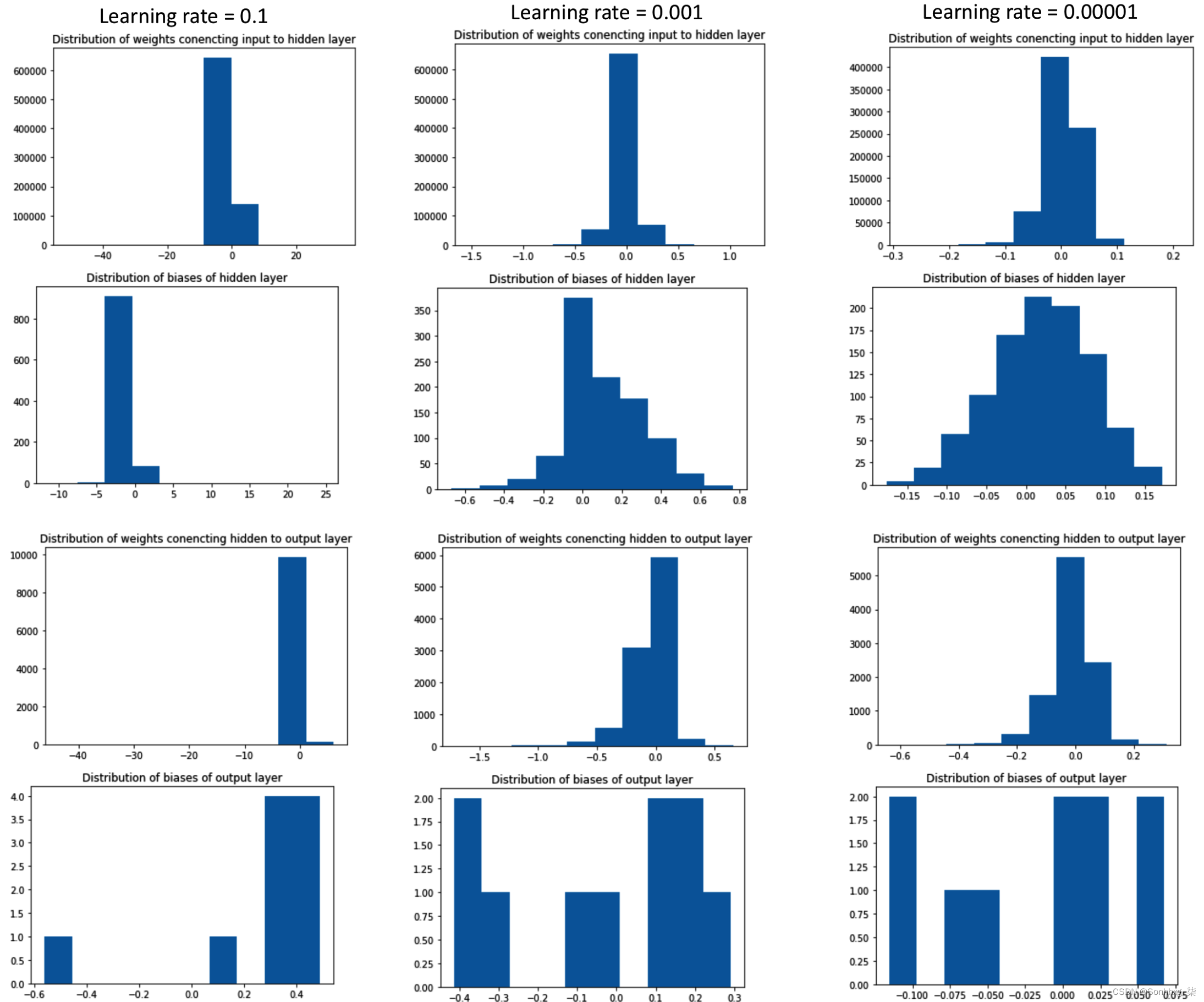• 当学习率高时，与中低学习率相比，参数的分布要大得多。
• 当参数分布较大时，就会发生过拟合。

## 改变学习率对非缩放数据集的影响

``````class FMNISTDataset(Dataset):
def __init__(self, x, y):
x = x.float() # Note that the data is not scaled in this
# scenario
x = x.view(-1,28*28)
self.x, self.y = x, y
def __getitem__(self, ix):
x, y = self.x[ix], self.y[ix]
return x.to(device), y.to(device)
def __len__(self):
return len(self.x)``````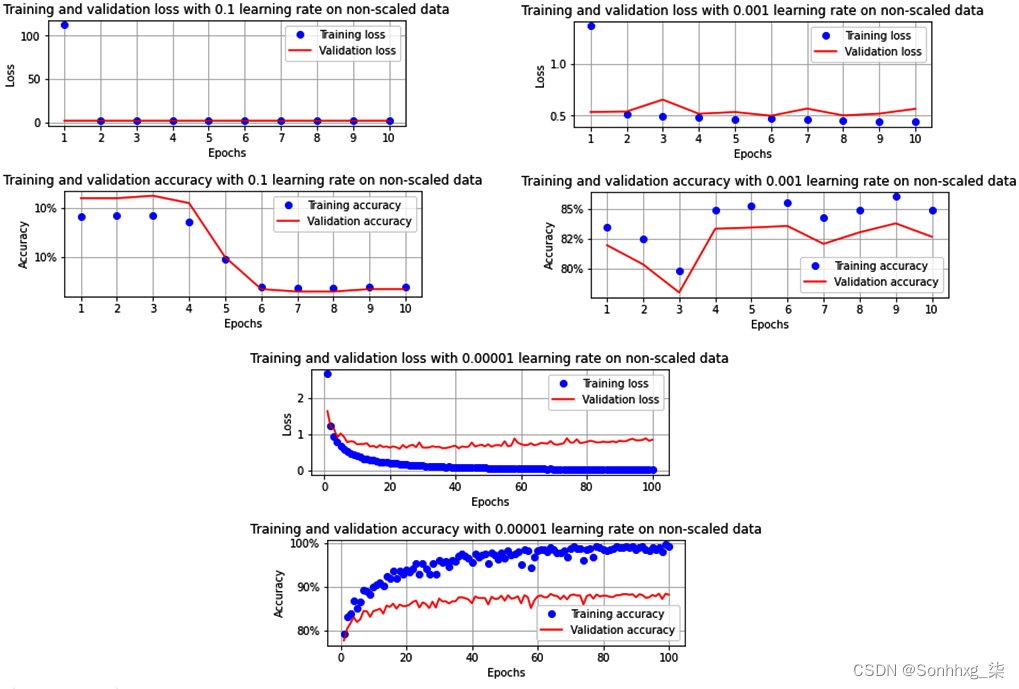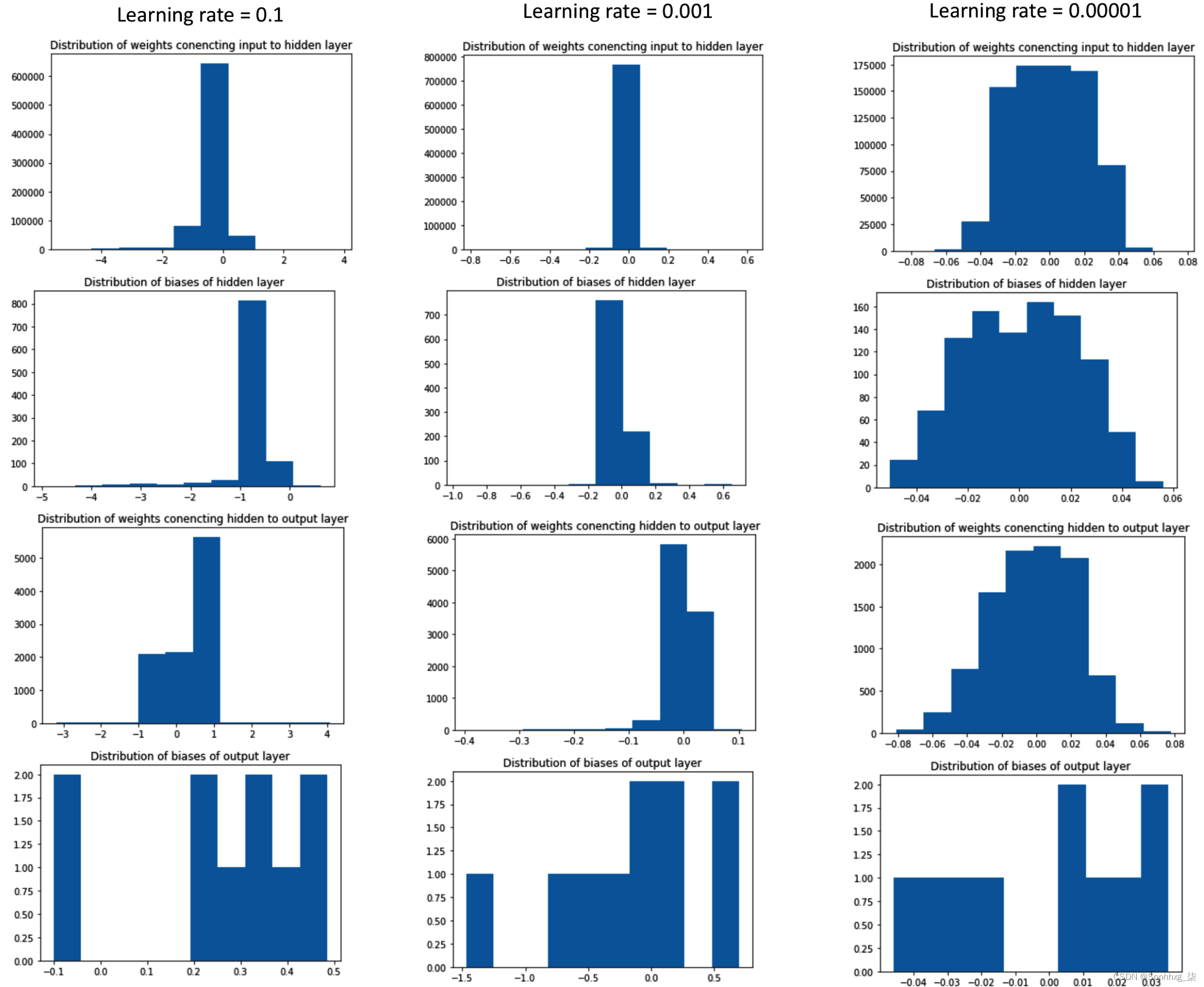# 了解学习率热启动的影响

PyTorch 为我们提供了工具，当验证损失在之前的“x”个时期没有减少时，我们可以使用这些工具来降低学习率。在这里，我们可以使用lr_scheduler方法：

``````from torch import optim
scheduler = optim.lr_scheduler.ReduceLROnPlateau(optimizer,
factor=0.5,patience=0,
threshold = 0.001,
verbose=True,
min_lr = 1e-5,
threshold_mode = 'abs')``````

``````from torch import optim
scheduler = optim.lr_scheduler.ReduceLROnPlateau(optimizer,
factor=0.5, patience=0,
threshold = 0.001,
verbose=True,
min_lr = 1e-5,
threshold_mode = 'abs')
train_losses, train_accuracies = [], []
val_losses, val_accuracies = [], []
for epoch in range(30):
#print(epoch)
train_epoch_losses, train_epoch_accuracies = [], []
for ix, batch in enumerate(iter(trn_dl)):
x, y = batch
batch_loss = train_batch(x, y, model, optimizer, \
loss_fn)
train_epoch_losses.append(batch_loss)
train_epoch_loss = np.array(train_epoch_losses).mean()

for ix, batch in enumerate(iter(trn_dl)):
x, y = batch
is_correct = accuracy(x, y, model)
train_epoch_accuracies.extend(is_correct)
train_epoch_accuracy = np.mean(train_epoch_accuracies)

for ix, batch in enumerate(iter(val_dl)):
x, y = batch
val_is_correct = accuracy(x, y, model)
validation_loss = val_loss(x, y, model)
scheduler.step(validation_loss)
val_epoch_accuracy = np.mean(val_is_correct)

train_losses.append(train_epoch_loss)
train_accuracies.append(train_epoch_accuracy)
val_losses.append(validation_loss)
val_accuracies.append(val_epoch_accuracy)``````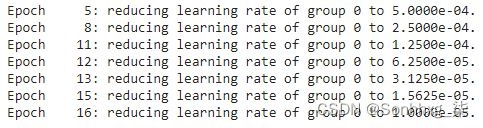让我们了解训练和验证数据集的准确性和损失值在增加的时期内的变化：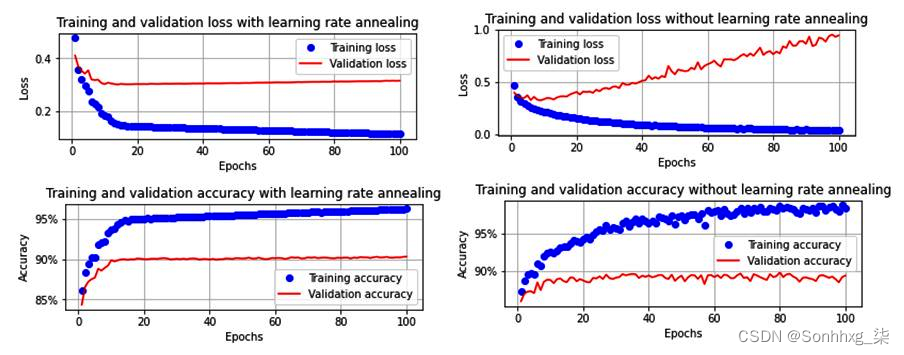# 构建更深层次的神经网络

``````def get_model():
model = nn.Sequential(
nn.Linear(28 * 28, 1000),
nn.ReLU(),
nn.Linear(1000, 1000),
nn.ReLU(),
nn.Linear(1000, 10)
).to(device)

loss_fn = nn.CrossEntropyLoss()
return model, loss_fn, optimizer``````
`类似地，没有隐藏层的get_model函数如下：`
``````def get_model():
model = nn.Sequential(
nn.Linear(28 * 28, 10)
).to(device)

loss_fn = nn.CrossEntropyLoss()
return model, loss_fn, optimizer``````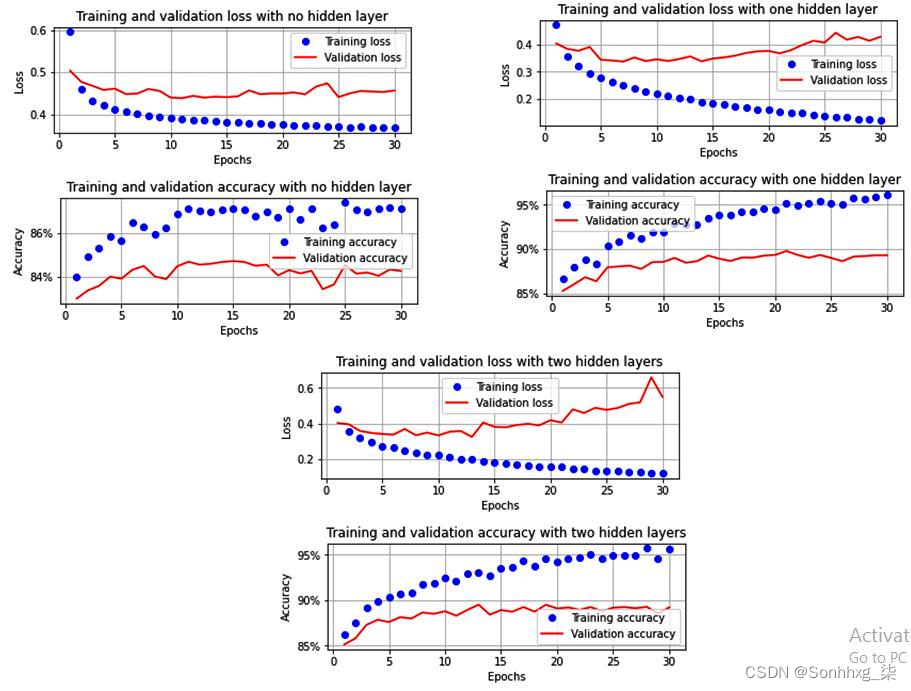• 当没有隐藏层时，模型无法学习。

• 与一个隐藏层相比，当有两个隐藏层时，模型的过度拟合量更大（与具有一层的模型相比，具有两层的模型中的验证损失更高）。

# 了解批量标准化的影响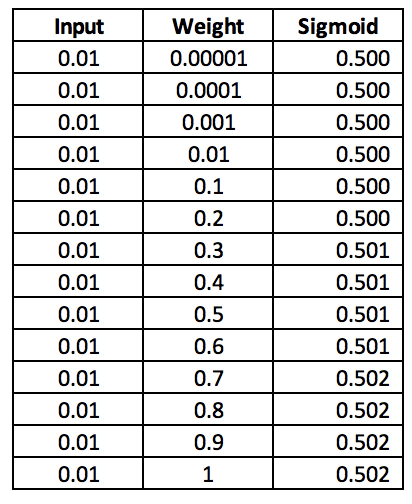通过从批次均值中减去每个数据点，然后将其除以批次方差，我们将一个节点处批次的所有数据点归一化到一个固定范围。

• 没有批量标准化的非常小的输入值
• 带有批量标准化的非常小的输入值

## 没有批量标准化的非常小的输入值

``````class FMNISTDataset(Dataset):
def __init__(self, x, y):
x = x.float()/(255*10000) # Done only for us to
# understand the impact of Batch normalization
x = x.view(-1,28*28)
self.x, self.y = x, y
def __getitem__(self, ix):
x, y = self.x[ix], self.y[ix]
return x.to(device), y.to(device)
def __len__(self):
return len(self.x)``````

``````def get_model():
class neuralnet(nn.Module):
def __init__(self):
super().__init__()
self.input_to_hidden_layer = nn.Linear(784,1000)
self.hidden_layer_activation = nn.ReLU()
self.hidden_to_output_layer = nn.Linear(1000,10)
def forward(self, x):
x = self.input_to_hidden_layer(x)
x1 = self.hidden_layer_activation(x)
x2= self.hidden_to_output_layer(x1)
return x2, x1
model = neuralnet().to(device)
loss_fn = nn.CrossEntropyLoss()
return model, loss_fn, optimizer``````

``````def train_batch(x, y, model, opt, loss_fn):
model.train()
prediction = model(x)
batch_loss = loss_fn(prediction, y)
batch_loss.backward()
optimizer.step()
return batch_loss.item()

def accuracy(x, y, model):
model.eval()
prediction = model(x)
max_values, argmaxes = prediction.max(-1)
is_correct = argmaxes == y
return is_correct.cpu().numpy().tolist()``````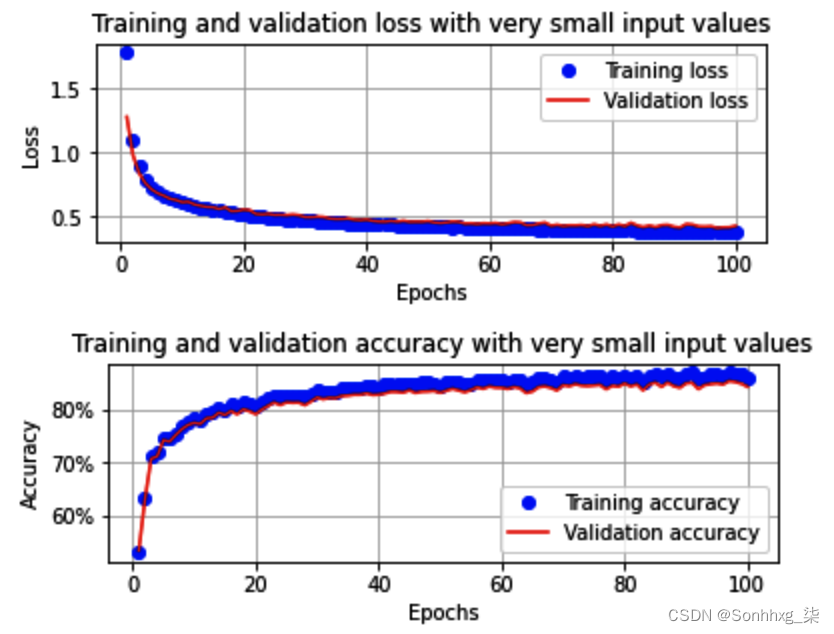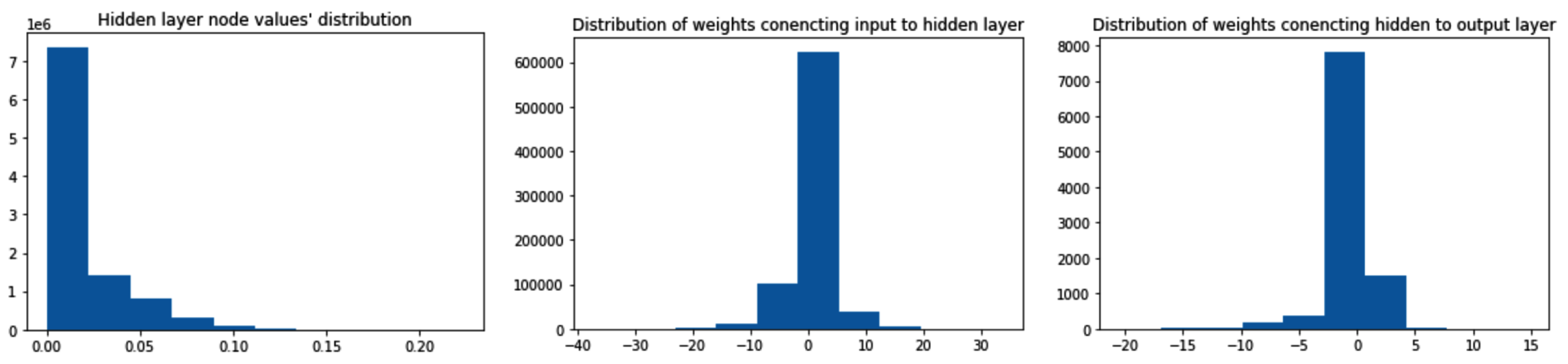## 带有批量标准化的非常小的输入值

``````def get_model():
class neuralnet(nn.Module):
def __init__(self):
super().__init__()
self.input_to_hidden_layer = nn.Linear(784,1000)
self.batch_norm = nn.BatchNorm1d(1000)
self.hidden_layer_activation = nn.ReLU()
self.hidden_to_output_layer = nn.Linear(1000,10)
def forward(self, x):
x = self.input_to_hidden_layer(x)
x0 = self.batch_norm(x)
x1 = self.hidden_layer_activation(x0)
x2= self.hidden_to_output_layer(x1)
return x2, x1
model = neuralnet().to(device)
loss_fn = nn.CrossEntropyLoss()
return model, loss_fn, optimizer``````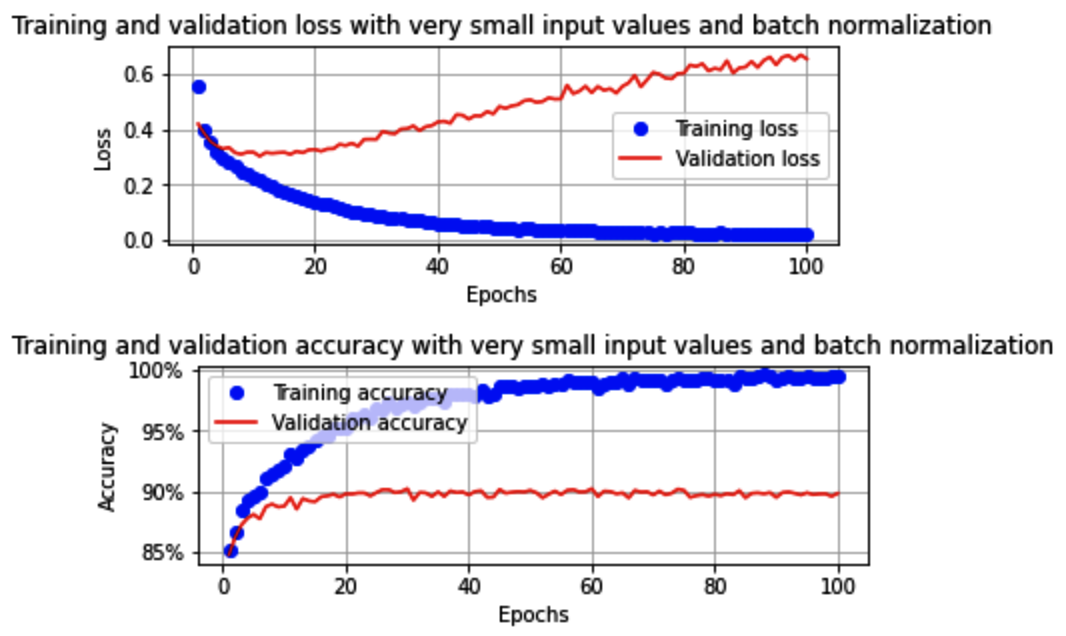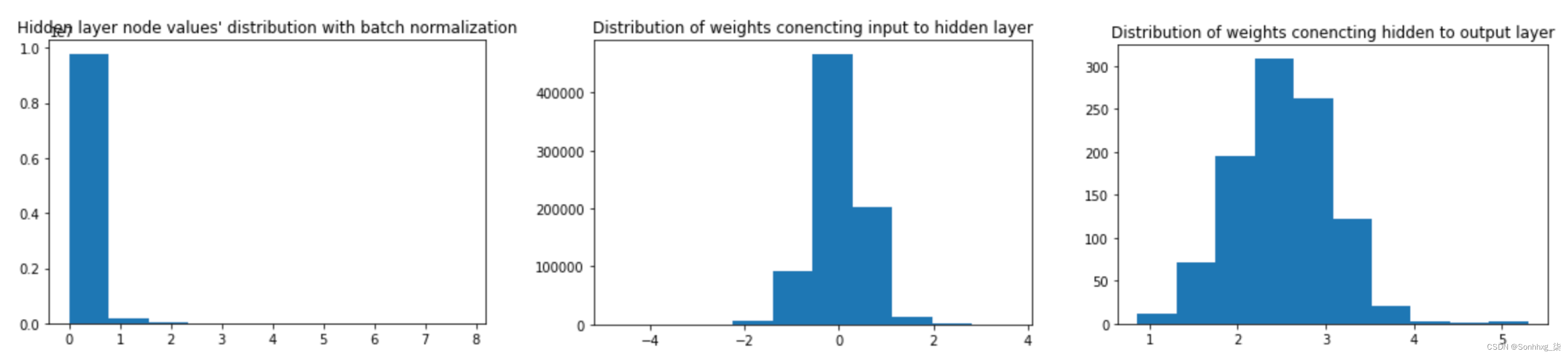# 过拟合的概念

• Dropout
• Regularization

## 添加 dropout 的影响

Dropout 是一种随机选择指定百分比的激活并将其降至 0 的机制。在下一次迭代中，另一组随机隐藏单元被关闭。这样，神经网络不会针对边缘情况进行优化，因为网络没有太多机会调整权重来记忆边缘情况（假设权重在每次迭代中都没有更新）。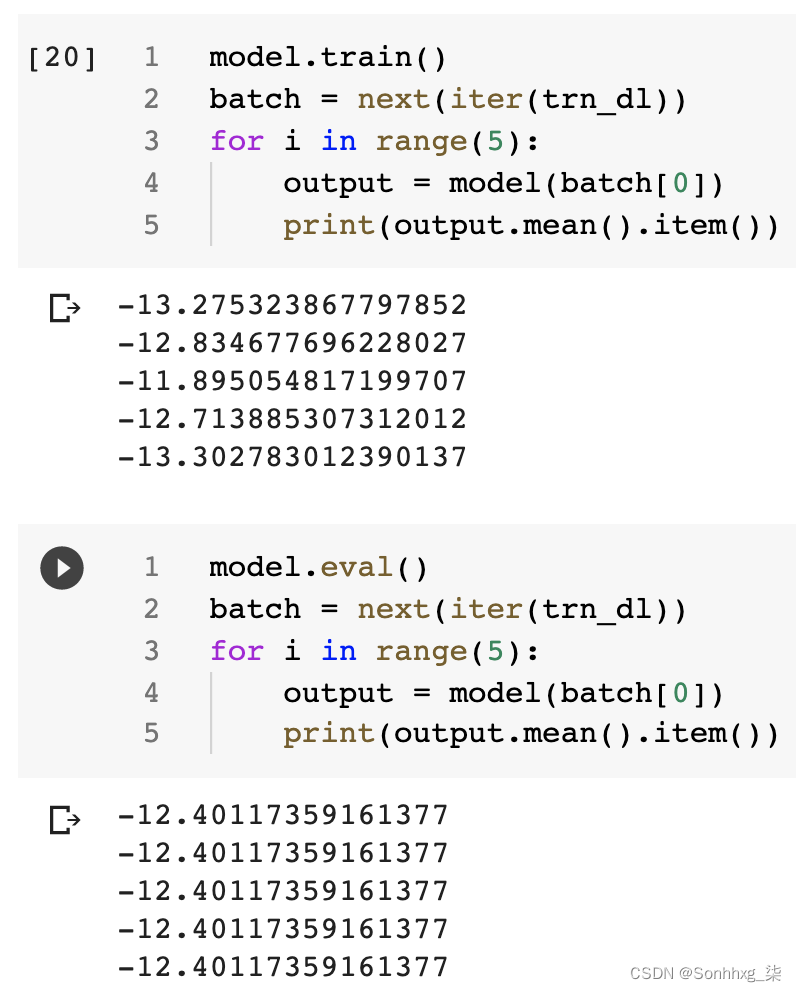``````def get_model():
model = nn.Sequential(
nn.Dropout(0.25),
nn.Linear(28 * 28, 1000),
nn.ReLU(),
nn.Dropout(0.25),
nn.Linear(1000, 10)
).to(device)

loss_fn = nn.CrossEntropyLoss()
return model, loss_fn, optimizer``````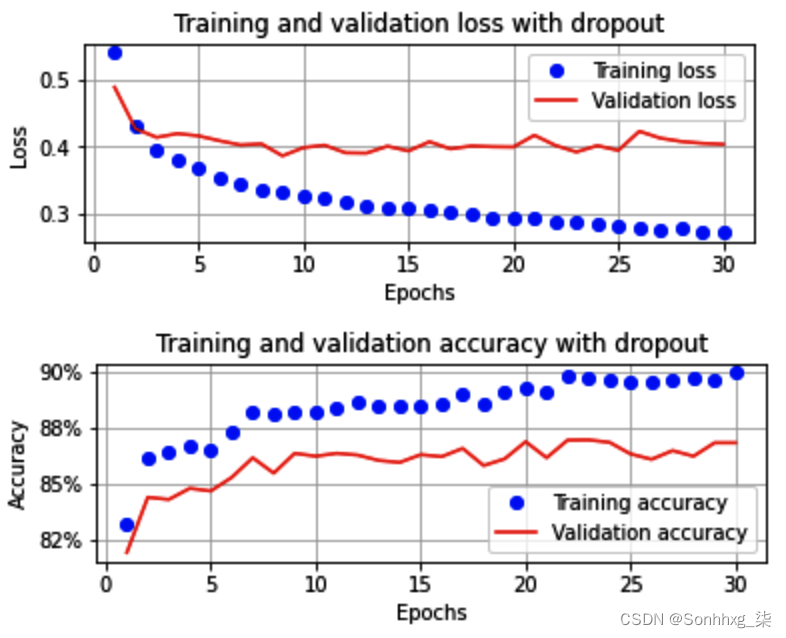## 正则化的影响

• L1 正则化
• L2 正则化

### L1 正则化

L1 正则化计算 如下：指的是我们与正则化（权重最小化）损失相关联的权重。

L1 正则化在训练模型的同时进行，如下：

``````def train_batch(x, y, model, opt, loss_fn):
model.train()
prediction = model(x)
l1_regularization = 0
for param in model.parameters():
l1_regularization += torch.norm(param,1)
batch_loss = loss_fn(prediction, y)+0.0001*l1_regularization
batch_loss.backward()
optimizer.step()
return batch_loss.item()``````

torch.norm(param,1) 提供跨层的权重和偏差值的绝对值。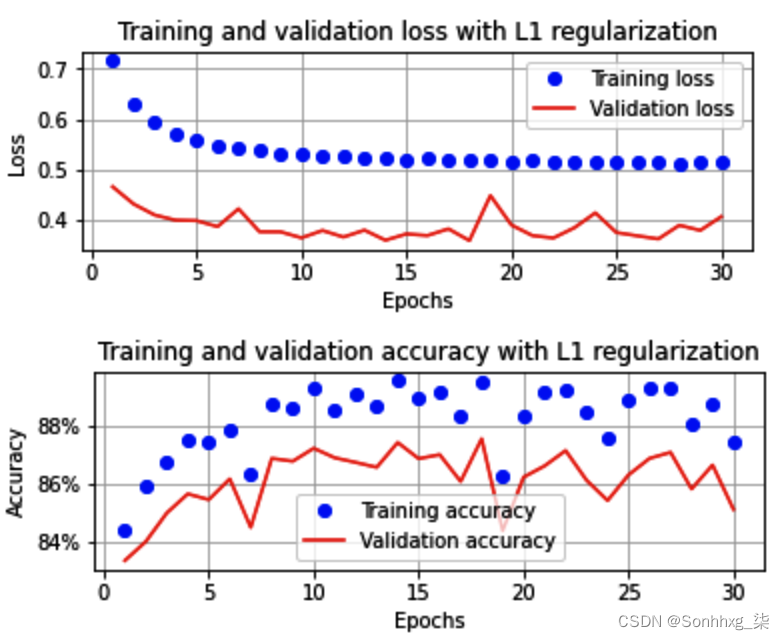### L2 正则化

L2正则化计算如下：上式的第一部分是指得到的分类交叉熵损失，而第二部分是指模型权重值的平方和。指的是我们与正则化（权重最小化）损失相关联的权重。

L2 正则化在训练模型时实现如下：

``````def train_batch(x, y, model, opt, loss_fn):
model.train()
prediction = model(x)
l2_regularization = 0
for param in model.parameters():
l2_regularization += torch.norm(param,2)
batch_loss = loss_fn(prediction, y) + 0.01*l2_regularization
batch_loss.backward()
optimizer.step()
return batch_loss.item()``````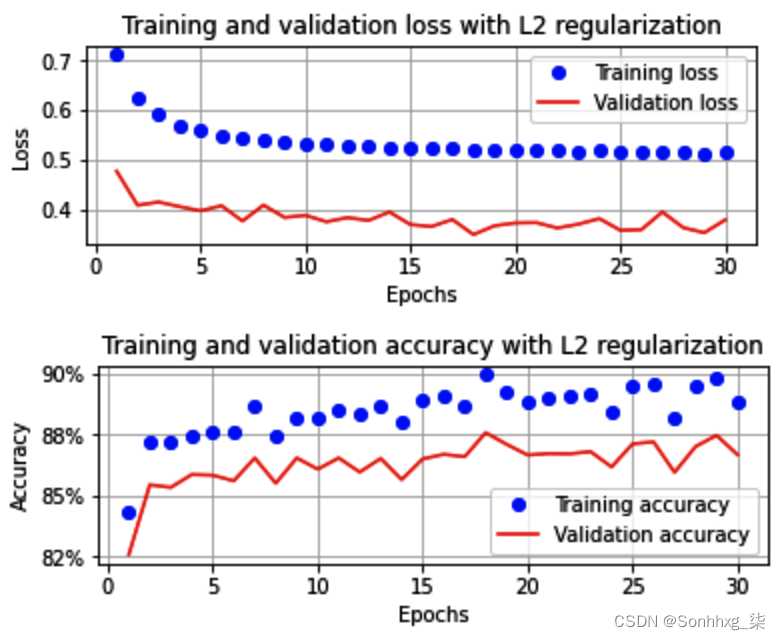在这里，我们可以看到 L2 正则化也导致验证和训练数据集的准确性和损失值彼此接近。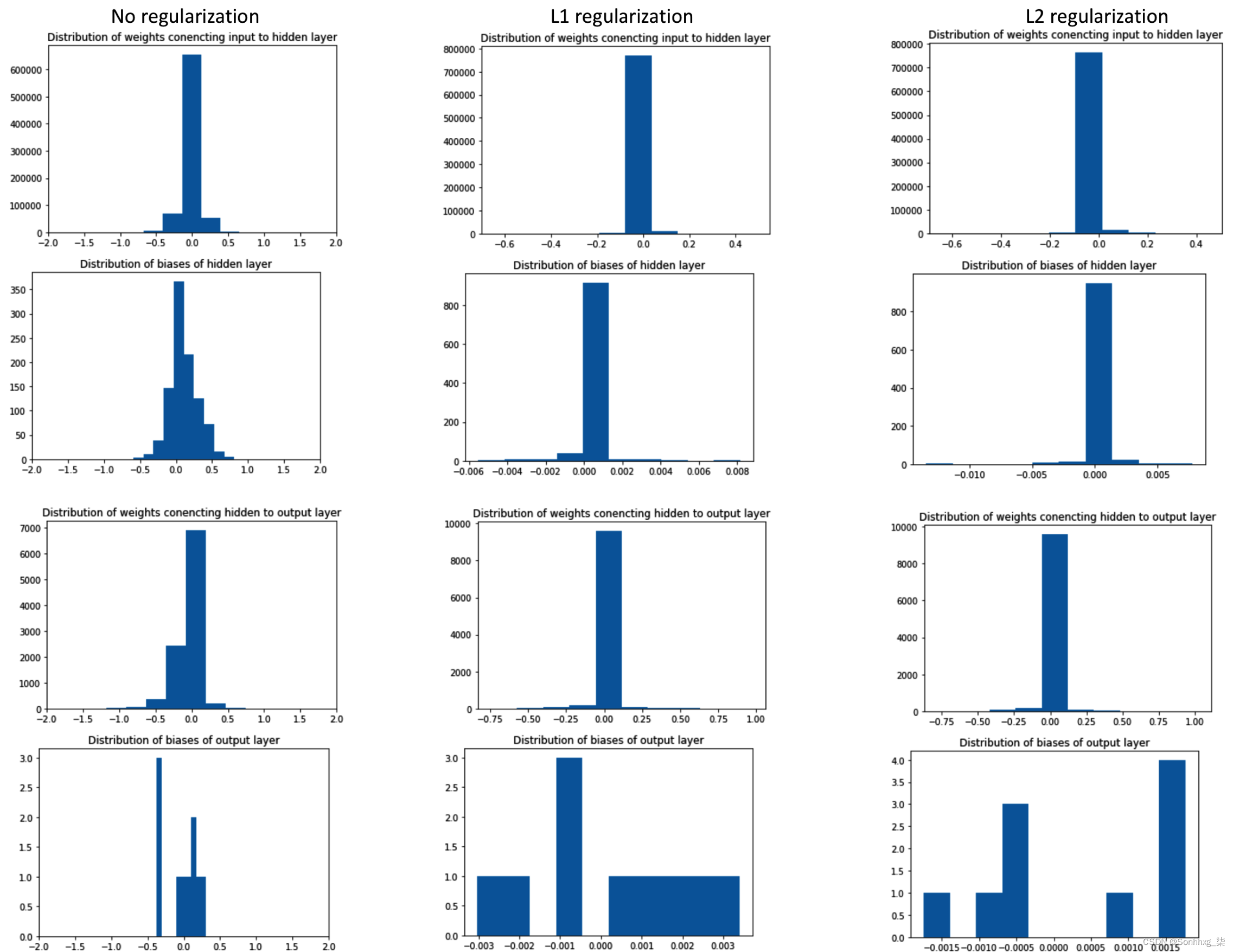# 问题

1. 如果输入值未在输入数据集中进行缩放，会发生什么情况？
2. 如果在训练神经网络时背景是白色像素颜色而内容是黑色像素颜色，会发生什么情况？
3. 批量大小对模型的训练时间以及给定数量的 epoch 的准确性有什么影响？
4. 输入值范围对训练结束时的权重分布有什么影响？
5. 批量标准化如何帮助提高准确性？
6. 我们如何知道模型是否在训练数据上过度拟合？
7. 正则化如何帮助避免过度拟合？
8. L1 和 L2 正则化有何不同？
9. dropout 如何帮助减少过拟合？